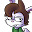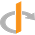# Erik McClure

Radians are used in almost everything that involves computers, and often come up in mathematical equations. However, most people are used to measuring angles in degrees, even though degrees (unlike radians) are a completely arbitrary measurement. To understand Radians, you need to understand $$\pi$$, or more precisely, why it sucks. Like a black hole, except black holes don't actually suck, they just warp space-time so you fall into them OK BACK ON TOPIC:

$$\pi$$ is the ratio of a circle's diameter to its circumference. This is retarded. When was the last time you thought of a circle using its diameter for crying out loud? We define circles, and should intuitively think of circles using their radius, which is the distance from the center to any point on the edge of the circle.

source: wikimedia commons

So that means that the ratio of the radius to the circumference is actually $$2\cdot\pi$$. This is why the formula for the circumference of a circle is $$2\cdot\pi\cdot r$$. In fact, its why we end up using $$2\cdot\pi$$ almost everywhere, because we almost always define circles with the radius, but $$\pi$$ is in relation to the diameter. Which is just stupid, and is in fact so stupid that some people are currently trying to define $$\tau$$ (Tau) such that $$\tau = 2\cdot\pi$$. Sadly, $$2\pi$$ still rules the world. I guess mathematicians really like pie…?

Either way, we must work within our constaints. So, keeping in mind that $$2\pi$$ is the ratio between a circle's circumference and its radius, what happens if you have a unit circle (a circle with a radius of 1)? Well, that means it has a diameter of 2, so if you roll it out on the number line, you get this:

When we talk about rotation and angles in radians, we are using the following relation:

$360^{\circ} = 2\pi\;rad$
That is, in radians, or $$rad$$ as it is commonly abbreviated, a full rotation is $$2\pi$$ because that's the circumference of a circle with a radius of 1. Radius → Radians. We know why its $$2\pi$$ instead of just $$pi$$, because $$pi$$ is stupid. If you want, you can say that $$\tau = 2\pi$$, so that $$\tau$$ is then equal to the ratio between a circle's radius and its circumference. Then we get the much nicer relation:
$360^{\circ} = \tau\;rad$
360° is just one rotation of a circle. A radian is simply how far around the circumference of a unit circle you want to go. So that means 180° is halfway around the circle, which is $$\tau/2$$ or just $$\pi$$ radians.
360°$$2\pi$$
180°$$\pi$$
90°$$\frac{\pi}{2}$$
60°$$\frac{\pi}{3}$$
45°$$\frac{\pi}{4}$$
30°$$\frac{\pi}{6}$$
This gives rise to the conversion functions:
\begin{aligned} rad = deg \cdot \left(\frac{\pi}{180}\right) \\ deg = rad \cdot \left(\frac{180}{\pi}\right) \end{aligned}
But these simply arise out of $$2\pi$$ being simplified:
\begin{aligned} rad = deg \cdot \left(\frac{2\pi}{360}\right) \\ deg = rad \cdot \left(\frac{360}{2\pi}\right) \end{aligned}
So now we have an intuitive explanation of what radians are - the distance around the circumference of the unit circle.tbushell

"When was the last time you thought of a circle using its diameter for crying out loud?"

Only ALL THE TIME - every time I drill a hole, or buy some pipe or tubing, or use a nut or a bolt, etc, etc, etc

So I have a little problem with your basic premise... ;-)

Nice animation, however.

Since I'm currently working on someone else's C# code that has stupid degree-to-radian conversions all through it - I'd like to know why modern languages don't just do everything in degrees.

-Tom BushellErik McClure

The purpose of this is mostly to explain to people why its 2 pi and not just pi, which is a major stumbling block to some. I've since reversed my opinion on whether or not it should be pi or tau, but it is still used here for the purposes of education.

As for why we don't do everything in degrees, its usually because all the math works with radians. Even if you did things in degrees, at some level you would still have to convert to radians and back again, because radians is what the actual math works with. It's also a matter of personal preference - some people like degrees, others like radians. Sometimes the preference changes depending on the context. You can *actually do this* with C++11's new user-defined literals. You could define a degree literal and it would automatically convert degrees to radians for use in functions, so you could put in, say 90deg, and it would be converted to pi/2.John

This comment has been removed by a blog administrator.